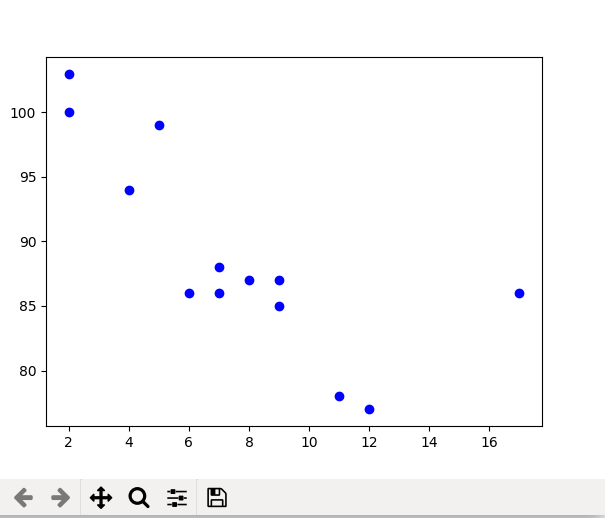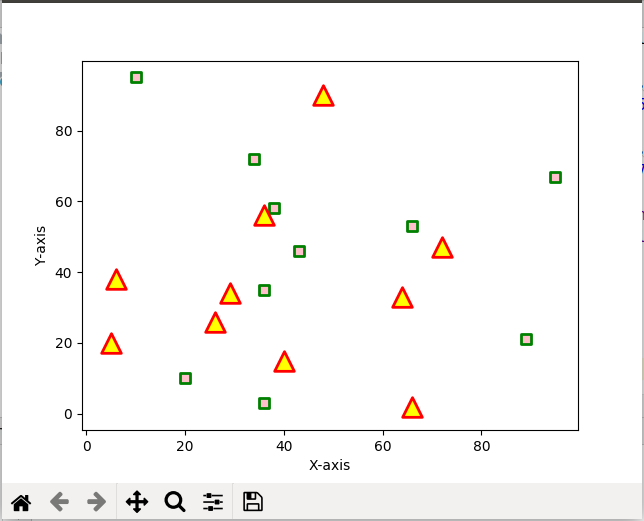# matplotlib.pyplot.scatter() in Python

• Last Updated : 15 Feb, 2022

Matplotlib is a comprehensive library for creating static, animated, and interactive visualizations in Python. It is used for plotting various plots in Python like scatter plot, bar charts, pie charts, line plots, histograms, 3-D plots and many more. We will learn about the scatter plot from the matplotlib library.
Note: For more information, refer to Python Matplotlib – An Overview

## matplotlib.pyplot.scatter()

Scatter plots are used to observe relationship between variables and uses dots to represent the relationship between them. The scatter() method in the matplotlib library is used to draw a scatter plot. Scatter plots are widely used to represent relation among variables and how change in one affects the other.
Syntax
The syntax for scatter() method is given below:

matplotlib.pyplot.scatter(x_axis_data, y_axis_data, s=None, c=None, marker=None, cmap=None, vmin=None, vmax=None, alpha=None, linewidths=None, edgecolors=None)

The scatter() method takes in the following parameters:

• x_axis_data- An array containing x-axis data
• y_axis_data- An array containing y-axis data
• s- marker size (can be scalar or array of size equal to size of x or y)
• c- color of sequence of colors for markers
• marker- marker style
• cmap- cmap name
• linewidths- width of marker border
• edgecolor- marker border color
• alpha- blending value, between 0 (transparent) and 1 (opaque)

Except x_axis_data and y_axis_data all other parameters are optional and their default value is None. Below are the scatter plot examples with various parameters.
Example 1: This is the most basic example of a scatter plot.

## Python3

 `import` `matplotlib.pyplot as plt`  `x ``=``[``5``, ``7``, ``8``, ``7``, ``2``, ``17``, ``2``, ``9``,``    ``4``, ``11``, ``12``, ``9``, ``6``]` `y ``=``[``99``, ``86``, ``87``, ``88``, ``100``, ``86``,``    ``103``, ``87``, ``94``, ``78``, ``77``, ``85``, ``86``]` `plt.scatter(x, y, c ``=``"blue"``)` `# To show the plot``plt.show()`

OutputExample 2: Scatter plot with different shape and colour for two datasets.

## Python3

 `import` `matplotlib.pyplot as plt` `# dataset-1``x1 ``=` `[``89``, ``43``, ``36``, ``36``, ``95``, ``10``,``      ``66``, ``34``, ``38``, ``20``]` `y1 ``=` `[``21``, ``46``, ``3``, ``35``, ``67``, ``95``,``      ``53``, ``72``, ``58``, ``10``]` `# dataset2``x2 ``=` `[``26``, ``29``, ``48``, ``64``, ``6``, ``5``,``      ``36``, ``66``, ``72``, ``40``]` `y2 ``=` `[``26``, ``34``, ``90``, ``33``, ``38``,``      ``20``, ``56``, ``2``, ``47``, ``15``]` `plt.scatter(x1, y1, c ``=``"pink"``,``            ``linewidths ``=` `2``,``            ``marker ``=``"s"``,``            ``edgecolor ``=``"green"``,``            ``s ``=` `50``)` `plt.scatter(x2, y2, c ``=``"yellow"``,``            ``linewidths ``=` `2``,``            ``marker ``=``"^"``,``            ``edgecolor ``=``"red"``,``            ``s ``=` `200``)` `plt.xlabel(``"X-axis"``)``plt.ylabel(``"Y-axis"``)``plt.show()`

OutputMy Personal Notes arrow_drop_up